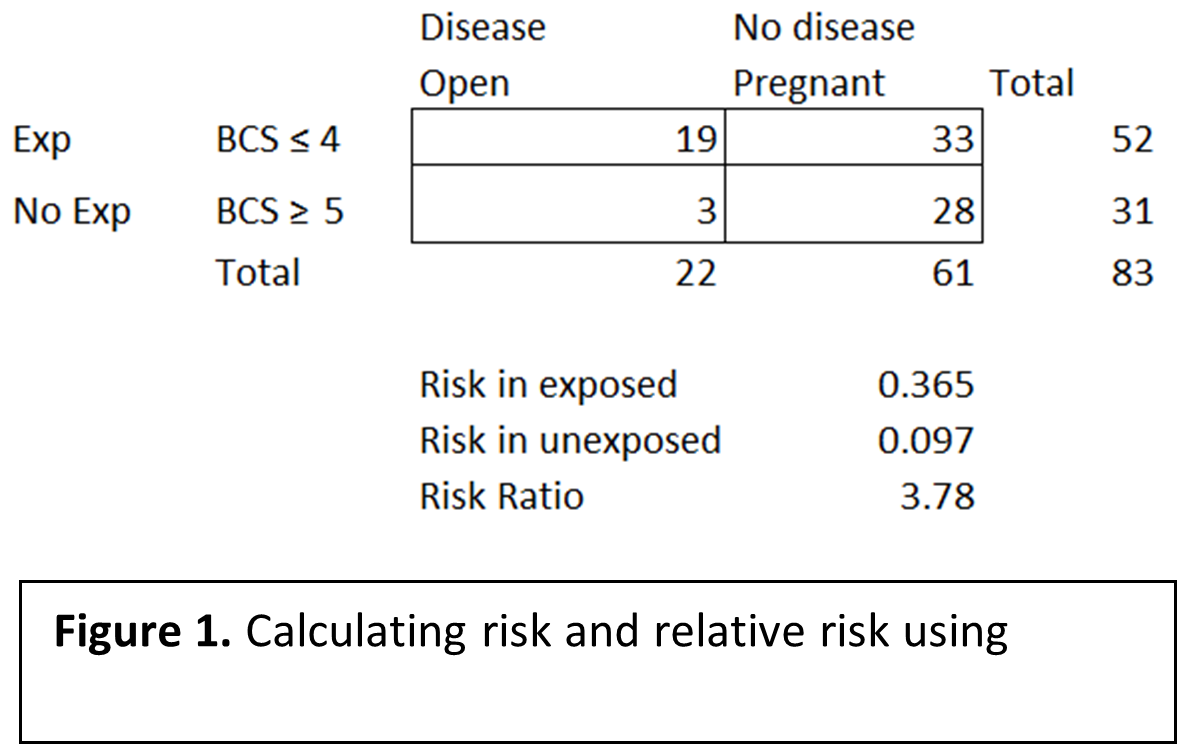# Measuring risk

When investigating problems of illness, death, or decreased production in cow-calf herds, a systematic approach to defining the problem, identifying risk factors, developing a plan, measuring impacts, and communicating results can aid the veterinarian and their client in resolving the problem. Once the problem has been properly defined (see previous articles regarding “defining the problem”) and a case definition has been established, the next step is identification and analysis of risk factors that can be manipulated to affect the problem.

Identifying risk factors for the outcome of interest (i.e. disease or decreased production) is the primary goal of epidemiologic investigations. These risk factors are sometimes called key determinants, and are often leverage points within the production system that can be manipulated to impact the outcome of interest. The veterinary practitioner’s goal is to identify leverage points that have a large positive impact on the problem, are economically and practically achievable, and can be measured. Data is the most useful tool when attempting to identify these key determinants. Data describing the outbreak such as animal age, when treatments occurred, when disease was noted, what treatments were used, location when disease occurred, management differences among cases and controls, etc. are valuable in outbreak investigations. Measures of association (e.g. risk ratios) can be used to measure the association between a risk factor and the outcome of interest (i.e. disease, decreased production, or any other issue of interest).

Figure 1 displays an example of using risk ratios, also known as relative risk, to measure the strength of association between cow body condition and pregnancy rates. Note that the data needed for these calculations includes only whether the cow was pregnant or open, and a body condition score (BCS) of 1-9, both of which can be easily recorded when performing pregnancy examinations. Risk ratios are ratios of the risk in the exposed group (i.e. cows with a BCS of less than or equal to 4) to the risk in the unexposed group (i.e. cows in adequate BCS, or BCS of greater than or equal to 5). Risk in each of the exposed and unexposed group is calculated by dividing the number of diseased individuals by the total number of individuals in each group. The risk ratio is calculated by dividing risk in exposed (i.e. 0.365) by risk in unexposed (i.e. 0.097). A risk ratio of greater than 1 means those exposed individuals are more likely to develop the “disease” (i.e. open in the example above) compared to the unexposed. A risk ratio of less than 1 means the exposure is protective (i.e. exposed individuals are less likely to develop the “disease”), and a risk ratio of 1 indicates no difference in risk of disease in either the exposed or unexposed groups. In Figure 1, the risk ratio of 3.78 indicates the cows in a BCS ≤ 4 are 3.78 times more likely to be open than cows in a BCS ≥ 5.In this example, the veterinarian and the producer could address body condition as a risk factor contributing to the number of open cows.